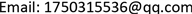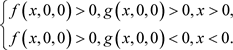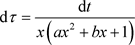﻿ Leslie型捕食者–食饵系统的弛豫振荡分析 Relaxation Oscillations Analysis in a Predator-Prey System of Leslie Type

Vol. 08  No. 12 ( 2019 ), Article ID: 33352 , 6 pages
10.12677/AAM.2019.812222

Relaxation Oscillations Analysis in a Predator-Prey System of Leslie Type

Chenrong Pan, Songlin Chen

School of Mathematical Science and Engineering, Anhui University of Technology, Ma’anshan AnhuiReceived: Nov. 9th, 2019; accepted: Dec. 2nd, 2019; published: Dec. 9th, 2019ABSTRACT

In this paper, we consider a predator-prey system of Leslie type with functional response. The equilibrium point type and stability of the system are analyzed, and the existence and uniqueness of relaxation oscillation period of the system are proved by using the entry-exit function and geometric singular perturbation theory.

Keywords:Leslie Predator-Prey System, Entry-Exit Function, Geometric Singular Perturbation Theory, Existence and Uniqueness

Leslie型捕食者–食饵系统的弛豫振荡分析1. 引言

$\left\{\begin{array}{l}\frac{\text{d}x}{\text{d}t}=rx\left(1-\frac{x}{K}\right)-\frac{mxy}{a{x}^{2}+bx+1},\\ \frac{\text{d}y}{\text{d}t}=sy\left(1-\frac{y}{hx}\right).\end{array}$ (1)

$\stackrel{¯}{t}=rt,\stackrel{¯}{x}=\frac{x}{K},\stackrel{¯}{y}=\frac{my}{r},\stackrel{¯}{a}=a{K}^{2},\stackrel{¯}{b}=bK,\epsilon =\frac{s}{r},\beta =\frac{r}{hmK}.$ (2)

$\stackrel{¯}{x},\stackrel{¯}{y},\stackrel{¯}{t},\stackrel{¯}{a},\stackrel{¯}{b}$ 仍记为 $x,y,t,a,b$，则系统(1)可以化为：

$\left\{\begin{array}{l}\frac{\text{d}x}{\text{d}t}=x\left(1-x\right)-\frac{xy}{a{x}^{2}+bx+1},\\ \frac{\text{d}y}{\text{d}t}=\epsilon y\left(1-\frac{\beta y}{x}\right).\end{array}$ (3)

2. 研究背景

2.1. 系统(3)的平衡点分析

1) 如果 $\stackrel{¯}{\Delta }<0$，则系统(3)有一个基本的正平衡点；

2) 如果 $\stackrel{¯}{\Delta }=0$，并且

2.1) ${\Delta }_{1}>0$，则系统(3)有两个不同的正平衡点：一个是基本的正平衡点，另一个是退化的正平衡点；

2.2) ${\Delta }_{1}=0$，则系统(3)有一个正平衡点，并且为退化的正平衡点；

3)如果 $\stackrel{¯}{\Delta }>0$，且 $b<\mathrm{min}\left\{a,\frac{1}{\beta }+1\right\}$，则系统(3)有三个不同的基本的正平衡点。

2.2. 入–出函数

$\left\{\begin{array}{l}\frac{\text{d}x}{\text{d}t}=\epsilon f\left(x,y,\epsilon \right),\\ \frac{\text{d}y}{\text{d}t}=g\left(x,y,\epsilon \right){y}^{2}.\end{array}$ (4)(5)

$\epsilon =\text{0}$ 时，解从点 $\left({x}_{0},{y}_{0}\right)$ 开始，其中， ${x}_{0}<0,{y}_{0}>0$，解快速地被吸引到x轴，然后沿着x轴向右漂移，最后被x轴排斥。它在x轴上的一点 ${p}_{\epsilon }\left({x}_{0}\right)$ 重新相交于直线 $y={y}_{0}$。当 $\epsilon \to \text{0}$ 时， ${p}_{\epsilon }\left({x}_{0}\right)\to {p}_{0}\left({x}_{0}\right)$。其中 ${p}_{0}\left({x}_{0}\right)$ 由下面的公式决定。

${\int }_{{x}_{0}}^{{p}_{0}\left({x}_{0}\right)}\frac{g\left(x,0,0\right)}{f\left(x,0,0\right)}\text{d}x=0.$ (6)

2.3. 极限系统的动力学

$\left\{\begin{array}{l}\epsilon \frac{\text{d}x}{\text{d}{t}_{1}}=x\left(1-x\right)-\frac{xy}{a{x}^{2}+bx+1},\\ \frac{\text{d}y}{\text{d}{t}_{1}}=y\left(1-\frac{\beta y}{x}\right).\end{array}$ (7)，当 $b>-2\sqrt{a}$ 时，有 $x\left(a{x}^{2}+bx+1\right)>0$。从而得到：

$\left\{\begin{array}{l}\epsilon \frac{\text{d}x}{\text{d}\tau }={x}^{2}\left(\left(1-x\right)\left(a{x}^{2}+bx+1\right)-y\right),\\ \frac{\text{d}y}{\text{d}\tau }=y\left(a{x}^{2}+bx+1\right)\left(x-\beta y\right).\end{array}$ (8)

$\left\{\begin{array}{l}\frac{\text{d}x}{\text{d}t}={x}^{2}\left(\left(1-x\right)\left(a{x}^{2}+bx+1\right)-y\right),\\ \frac{\text{d}y}{\text{d}t}=\epsilon y\left(a{x}^{2}+bx+1\right)\left(x-\beta y\right).\end{array}$ (9)

$\left\{\begin{array}{l}0={x}^{2}\left(\left(1-x\right)\left(a{x}^{2}+bx+1\right)-y\right),\\ \frac{\text{d}y}{\text{d}\tau }=y\left(a{x}^{2}+bx+1\right)\left(x-\beta y\right).\end{array}$ (10)

$\left\{\begin{array}{l}\frac{\text{d}x}{\text{d}t}={x}^{2}\left(\left(1-x\right)\left(a{x}^{2}+bx+1\right)-y\right),\\ \frac{\text{d}y}{\text{d}t}=\text{0}.\end{array}$ (11)

${C}_{0}=\left\{\left(x,y\right)|y=f\left(x\right)=\left(1-x\right)\left(a{x}^{2}+bx+1\right)\right\}.$ (12)

3. 弛豫振荡

${h}_{1}\left(x,y\right)=\left(1-x\right)\left(a{x}^{2}+bx+1\right)-y$${h}_{2}\left(x,y\right)=y\left(a{x}^{2}+bx+1\right)\left(x-\beta y\right)$，则(9)变为：

$\left\{\begin{array}{l}\frac{\text{d}x}{\text{d}t}={x}^{2}{h}_{1}\left(x,y\right),\\ \frac{\text{d}y}{\text{d}t}=\epsilon {h}_{2}\left(x,y\right).\end{array}$ (13)

(b)当 ${y}_{0}\to 0$ 时，有 ${\int }_{{y}_{0}}^{{y}_{\mathrm{max}}}\frac{{h}_{1}\left(0,y\right)}{{h}_{2}\left(0,y\right)}\text{d}y\to -\infty$。其中， $\left({x}_{\mathrm{max}},{y}_{\mathrm{max}}\right)$ 为一般的折点， ${x}_{\mathrm{max}}=\frac{2\left(a-1\right)}{3a}$${y}_{\mathrm{max}}=f\left({x}_{\mathrm{max}}\right)$

${\int }_{{y}_{0}^{*}}^{{y}_{\mathrm{max}}}\frac{{h}_{1}\left(0,y\right)}{{h}_{2}\left(0,y\right)}\text{d}y=0.$ (14)

$I\left({y}_{0}^{*}\right):={\int }_{{y}_{0}^{*}}^{{y}_{\mathrm{max}}}\frac{{h}_{1}\left(0,y\right)}{{h}_{2}\left(0,y\right)}\text{d}y={\int }_{{y}_{0}^{*}}^{{y}_{\mathrm{max}}}\frac{1-y}{-\beta {y}^{2}}\text{d}y\to -\infty ,{y}_{0}^{*}\to {0}^{+},$ (15)

${I}^{\prime }\left({y}_{0}^{*}\right)=-\frac{1-y}{-\beta {y}^{2}}=\frac{1-y}{\beta {y}^{2}}>0.$ (16)

$I\left({y}_{0}^{*}\right)={\int }_{{y}_{0}^{*}}^{1}\frac{1-y}{-\beta {y}^{2}}\text{d}y+{\int }_{1}^{{y}_{\mathrm{max}}}\frac{1-y}{-\beta {y}^{2}}\text{d}y,$ (17)

$I\left(1\right)={\int }_{1}^{{y}_{\mathrm{max}}}\frac{1-y}{-\beta {y}^{2}}\text{d}y>0.$ (18)

${\int }_{{y}_{0}^{*}}^{{y}_{\mathrm{max}}}\frac{{h}_{1}\left(0,y\right)}{{h}_{2}\left(0,y\right)}\text{d}y=0.$

Relaxation Oscillations Analysis in a Predator-Prey System of Leslie Type[J]. 应用数学进展, 2019, 08(12): 1937-1942. https://doi.org/10.12677/AAM.2019.812222

1. 1. Huang, J.C., Ruan, S.G. and Song, J. (2014) Bifurcations in a Predator-Prey System of Leslie Type with Generalized Holling Type III Functional Response. Journal of Differential Equations, 257, 1721-1752.

2. 2. Dai, Y.F., Zhao, Y.L. and Sang, B. (2019) Four Limit Cycles in a Predator-Prey System of Leslie Type with Generalized Holling Type III Functional Response. Nonlinear Analysis: Real World Applications, 50, 218-239.

3. 3. 卜令杰, 窦霁虹, 刘萌萌, 邢伟. 一类三次系统极限环的存在唯一性[J]. 延安大学学报(自然科学版), 2014, 33(2): 1-5.

4. 4. Valls, C. (2019) Nonlinear Oscillations in the Modified Leslie-Gower Model. Nonlinear Analysis: Real World Applications, 51, 1-7.

5. 5. 林园, 高瑾. Lotka-Volterra竞争扩散系统连接边界平衡点和正平衡点行波解的存在性[J]. 教育教学论坛, 2019(27): 95-98.

6. 6. Maesschalck, P.D. and Schecter, S. (2016) The Entry-Exit Function and Geometric Singular Perturbation Theory. Journal of Differential Equations, 260, 6697-6715.
https://doi.org/10.1016/j.jde.2016.01.008

7. 7. Lee, M.G. and Tzavaras, A. (2017) Existence of Localizing Solutions in Plasticity via Geometric Singular Perturbation Theory. SIAM Journal on Applied Dynamical Systems, 16, 337-360.
https://doi.org/10.1137/16M1087308

8. 8. 王国俊. Lukasiewicz语义集上的紧Hausdorff拓扑[J]. 数学学报, 2002, 45(5): 919-924.

9. 9. Panazzolo, D. and Da Silva, P.R. (2017) Regularization of Discontinuous Foliations: Blowing up and Sliding Conditions via Fenichel Theory. Journal of Differential Equations, 263, 8362-8390.
https://doi.org/10.1016/j.jde.2017.08.042

10. 10. Krupa, M. and Szmolyan, P. (2001) Extending Geometric Singular Perturbation Theory to Nonhyperbolic Points-Fold and Canard Points in Two Dimensions. SIAM Journal on Mathematical Analysis, 33, 286-314.
https://doi.org/10.1137/S0036141099360919

11. 11. Krupa, M. and Szmolyan, P. (2001) Relaxation Oscillation and Canard Explosion. Journal of Differential Equations, 174, 312-368.
https://doi.org/10.1006/jdeq.2000.3929